### Home > CC4 > Chapter 10 > Lesson 10.1.3 > Problem10-58

10-58.
1. Rewrite each expression to an equivalent one with no parentheses or negative exponents. Homework Help ✎

1. (−3x)2

2. (3x)−2

3.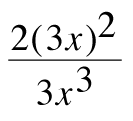4.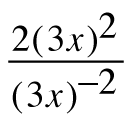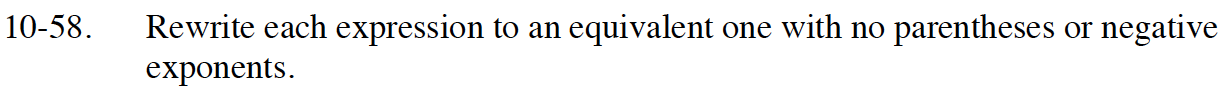Review the Math Notes box in section 1.3.2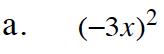9x2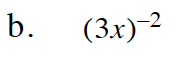$\frac{1}{(3x)^{2}}$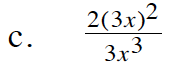$\frac{2(3x)(3x)}{3x^{3}}$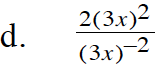$162x^{4}$